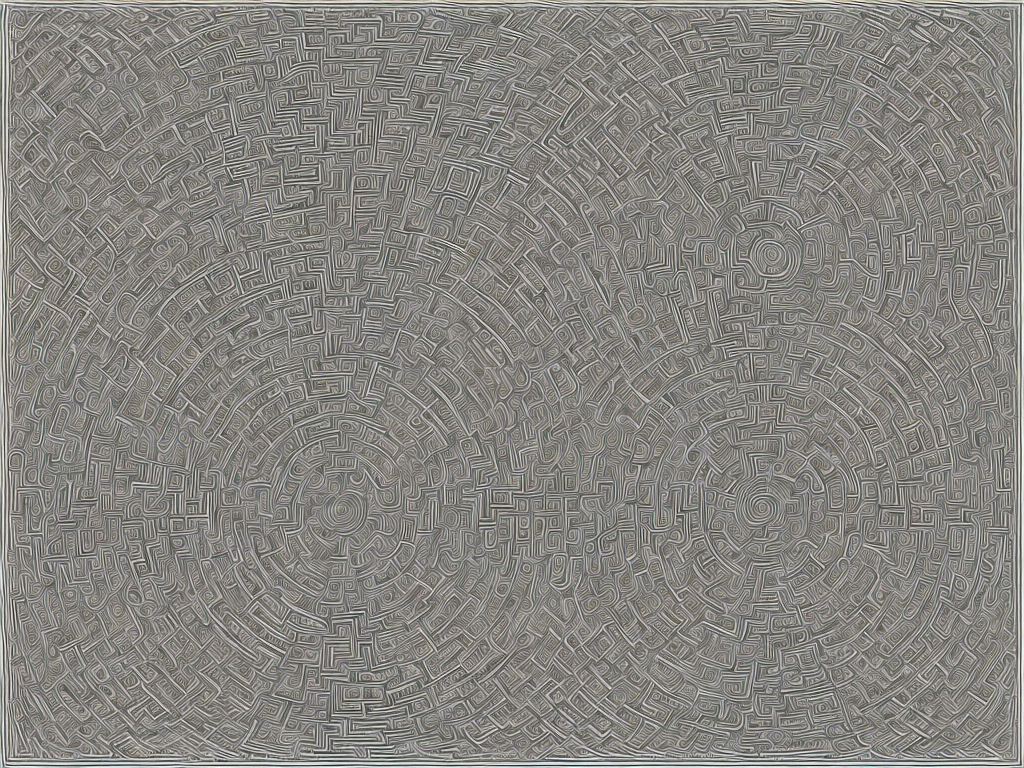# Difference Between Prims And Kruskal

tl;dr
Prims and Kruskal are two algorithms used to find the minimum spanning tree (MST) of a graph, with Prims selecting edges based on their weight and Kruskal sorting all edges and selecting them one by one to avoid cycles.Prims and Kruskal are two algorithms used in graph theory to find the minimum spanning tree (MST) of a given graph. A spanning tree is a tree that connects all vertices of the graph without forming a cycle. A minimum spanning tree is a tree that connects all vertices of the graph with the minimum possible cost.

The difference between Prims and Kruskal boils down to how they choose which edges to add to the minimum spanning tree. Prims algorithm starts with any vertex and adds the edge with the minimum weight connected to it at each step until all vertices are connected. Kruskal algorithm, on the other hand, sorts all the edges by weight and adds them to the tree one by one, skipping those that would create a cycle.

Prims Algorithm:

Prims algorithm is a greedy algorithm that starts with any arbitrary vertex and adds the next cheapest vertex to the MST. Here are the steps to use Prims algorithm:

1. Choose any vertex in the graph

2. Mark this vertex as visited and add all adjacent edges to a candidate edge list

3. Choose the minimum weight edge from the candidate list and mark it as visited

4. Add all the adjacent edges from the newly connected vertex to the candidate edge list

5. Repeat steps 3 and 4 until all vertices are included in the minimum spanning tree.

Prims algorithm works well for dense graphs (graphs with lots of edges) since it only considers edges adjacent to the current vertex. It has a complexity of O(n^2) and O(nlogn) with a heap.

Kruskal Algorithm:

Kruskal algorithm is a greedy algorithm that starts by sorting all edges and then chooses edges to be added to the minimum spanning tree based on their weight, while avoiding cycles. Here are the steps to use Kruskal algorithm:

1. Sort all edges based on their weight

2. Create a forest of all vertices, where each vertex is a separate tree

3. For each edge, add it to the tree if it does not create a cycle

4. Combine smaller trees into larger trees until all vertices are included in the MST.

Kruskal algorithm works efficiently for sparse graphs (graphs with fewer edges) since it sorts all the edges. It has a complexity of O(ElogE) and O(ElogV) with a heap.

Differences Between Prims And Kruskal:

1. Starting Point – Prims algorithm requires a starting vertex, while Kruskal algorithm does not. Prims algorithm starts with any arbitrary vertex, while Kruskal algorithms starts by sorting all edges.

2. Edge Selection – Prims algorithm selects edges based on their weight, while Kruskal algorithm sorts all edges and selects them one by one.

3. Data Structure – Prims algorithm uses a priority queue and a list to store candidate edges, while Kruskal algorithm uses a disjoint set data structure to detect cycles and store edges.

4. Complexity – Prims algorithm has a complexity of O(n^2) and O(nlogn) with a heap, while Kruskal algorithm has a complexity of O(ElogE) and O(ElogV) with a heap.

Conclusion:

In summary, both Prims and Kruskal algorithms are used to find the minimum spanning tree of a given graph. Prims algorithm chooses edges based on their weight, starting with any arbitrary vertex, and working towards all vertices. Kruskal algorithm, on the other hand, sorts all edges and selects them one by one, avoiding cycles and gradually connecting all the vertices. Both algorithms have their strengths and weaknesses, depending on the structure of the graph. Prims algorithm works well for dense graphs, while Kruskal algorithm works efficiently for sparse graphs.